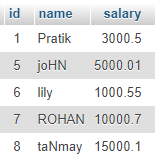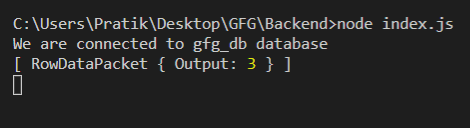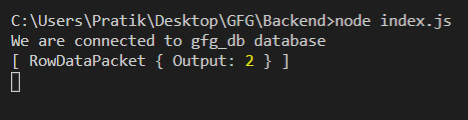GFG App
Open AppBrowser
Continue

# Node.js MySQL FIELD() Function

FIELD() function is a built-in function in MySQL that is used to get the position of the first occurrence of a value in a set of expressions. In the case of string values, it is not case-sensitive.

Syntax:

`FIELD(value, input_1, input_2, input_3, ...)`

Parameters: It takes two parameters as follows:

• value: It is the given value to be searched.
• input: It is the inputs that are checked for equality against the given value.

Return Value: It returns the position of the first occurrence of a value in a set of expressions. If nothing found then it will return 0.

Module Installation: Install the mysql module using the following command:

`npm install mysql`

Database: Our SQL publishers table preview with sample data is shown below:Example 1:

## index.js

 `const mysql = require(``"mysql"``); ` ` `  `let db_con  = mysql.createConnection({ ` `    ``host: ``"localhost"``, ` `    ``user: ``"root"``, ` `    ``password: ``''``, ` `    ``database: ``'gfg_db'` `}); ` ` `  `db_con.connect((err) => { ` `    ``if` `(err) { ` `      ``console.log(``"Database Connection Failed !!!"``, err); ` `      ``return``; ` `    ``} ` ` `  `    ``console.log(``"We are connected to gfg_db database"``); ` ` `  `    ``// Here is the query ` `    ``let query = ``"SELECT FIELD(2, 12, 15, 2, 122) AS Output"``; ` ` `  `    ``db_con.query(query, (err, rows) => { ` `        ``if``(err) ``throw` `err; ` ` `  `        ``console.log(rows); ` `    ``}); ` `});`

Run the index.js file using the following command:

`node index.js`

Output:Example 2:

## index.js

 `const mysql = require(``"mysql"``); ` ` `  `let db_con  = mysql.createConnection({ ` `    ``host: ``"localhost"``, ` `    ``user: ``"root"``, ` `    ``password: ``''``, ` `    ``database: ``'gfg_db'` `}); ` ` `  `db_con.connect((err) => { ` `    ``if` `(err) { ` `      ``console.log(``"Database Connection Failed !!!"``, err); ` `      ``return``; ` `    ``} ` ` `  `    ``console.log(``"We are connected to gfg_db database"``); ` ` `  `    ``// Here is the query ` `    ``let query =  ` `"SELECT FIELD('geek', 'Geeek', 'gEEK', 'geeK') AS Output"``; ` ` `  `    ``db_con.query(query, (err, rows) => { ` `        ``if``(err) ``throw` `err; ` ` `  `        ``console.log(rows); ` `    ``}); ` `});`

Run the index.js file using the following command:

`node index.js`

Output:My Personal Notes arrow_drop_up